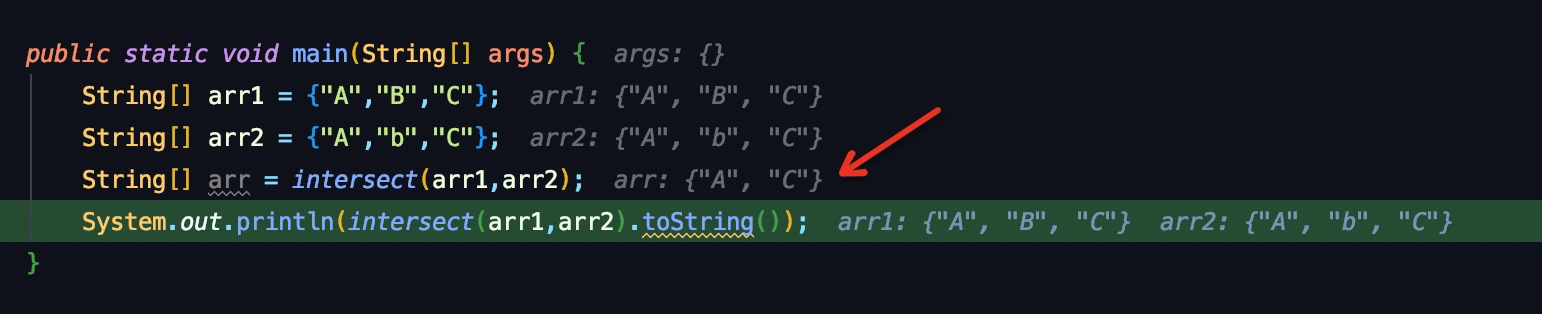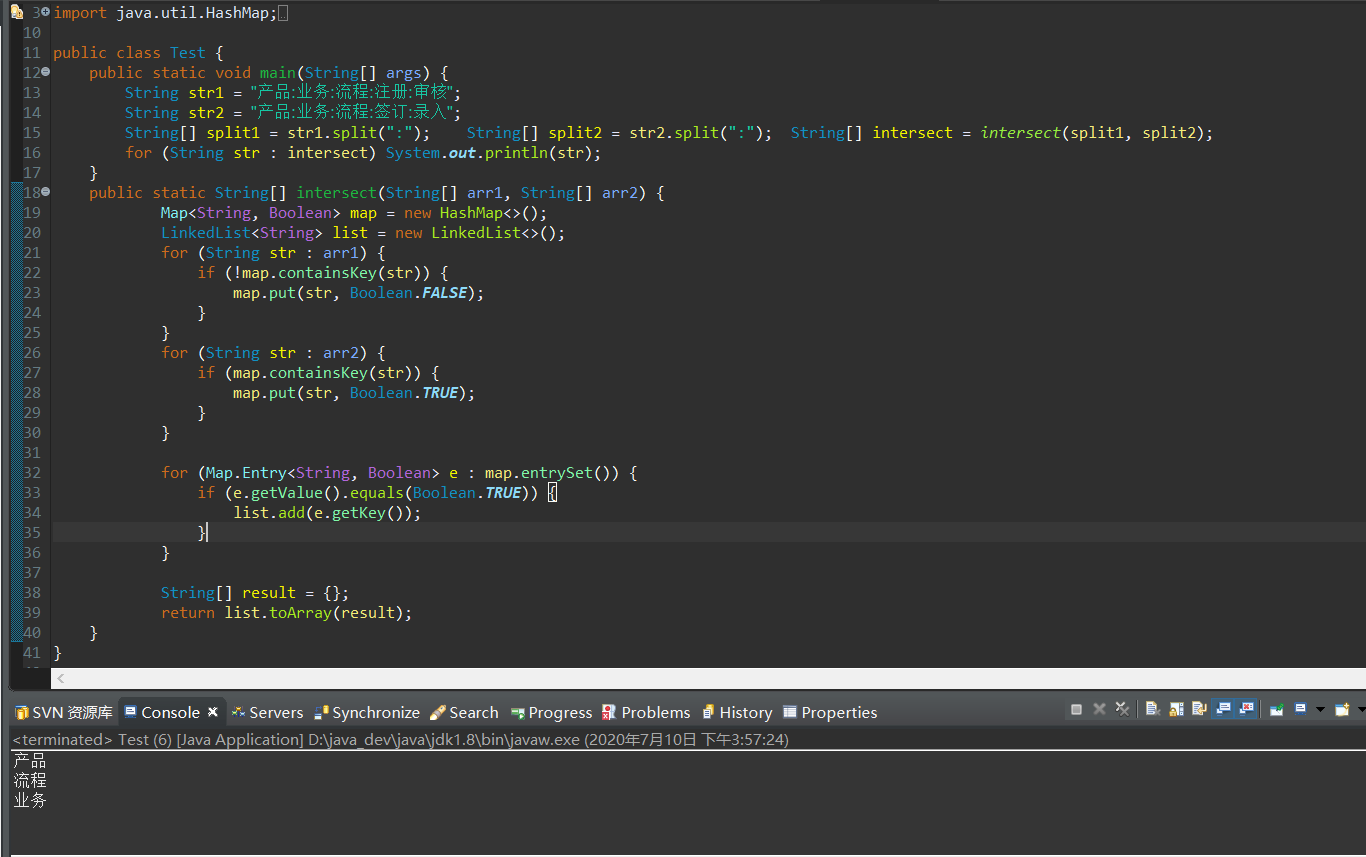• private static String[] intersect(String[] arr1, String[] arr2) { return Arrays.stream(arr1).filter(o -> Arrays.stream(arr2).anyMatch(str -> StringUtils.equals(str, o))).toArray(String[]::n...
private static String[] intersect(String[] arr1, String[] arr2) {
return Arrays.stream(arr1).filter(o -> Arrays.stream(arr2).anyMatch(str -> StringUtils.equals(str, o))).toArray(String[]::new);
}
测试正确：展开全文• 两个数组交集（力扣：349） 给定两个数组，编写一个函数来计算它们的交集。 说明： 输出结果中的每个元素一定是唯一的。 我们可以不考虑输出结果的顺序。
两个数组的交集

题目
两个数组的交集（力扣：349）
给定两个数组，编写一个函数来计算它们的交集。
说明：
输出结果中的每个元素一定是唯一的。 我们可以不考虑输出结果的顺序。
分析
本题的要点是：
找到交集元素。结果要去重。
方法一：使用Set的特性（不允许重复），把数组1保存在HashSet中，然后遍历数组2的值，如果当前值已存在Set中，则该值为交集。同理，我们将交集保存在Set中去重，最后转换成int数组返回。
方法二：遍历数组1，将int值的最小值和最大值边界找到；创建一个以最大值和最小值的差为大小的布尔数组，用于保存是否交集（true）表示交集。然后遍历数组2，当数组2中的值在数组1最大、最小值范围内，并且对应的布尔值为true，则保存，保存后将对应的布尔值置位false，来避免重复。
代码实现：方法一
    /**
* 349. 两个数组的交集
* @param nums1
* @param nums2
* @return
*/
public int[] intersection(int[] nums1, int[] nums2) {
Set<Integer> set1 = new HashSet<>();
for (int i=0;i<nums1.length;i++){
}
Set<Integer> resSet = new HashSet<>();

for (int j=0;j<nums2.length;j++){
if (set1.contains(nums2[j])){
}
}
int[] res = new int[resSet.size()];
int k = 0;
for (Integer v : resSet){
res[k] = v;
k++;
}
return res;
}

代码实现：方法二
    /**
* 349. 两个数组的交集
* @param nums1
* @param nums2
* @return
*/
public int[] intersection2(int[] nums1, int[] nums2) {
// 确定数组 nums1 的取值范围
int max = Integer.MIN_VALUE;
int min = Integer.MAX_VALUE;
for (int num : nums1) {
if (num > max) {
max = num;
}
if (num < min) {
min = num;
}
}
boolean[] arr = new boolean[max - min + 1];
for (int num : nums1) {
arr[num - min] = true;
}
// 判断数组 nums2 中的数是否在数组 nums1 中存在，
// 如果存在保存在数组 tmp 中
int[] tmp = new int[max - min + 1];
int idx = 0;
for (int num : nums2) {
if (num >= min && num <= max && arr[num - min]) {
tmp[idx++] = num;
arr[num- min] = false;//保证每个值只存储一次
}
}

// 修剪数组，返回结果
int[] ret = new int[idx];
for (int i = 0; i < idx; i++) {
ret[i] = tmp[i];
}
return ret;
}

测试结果：方法二的效率明显高于方法一。
展开全文leetcode 算法
• 给定两个排序后的数组，编写一个Java代码来查找两个数组交集。 例如，如果输入数组是： arr1 [] = {2，3，6，7，9，11} arr2 [] = {4，6，8，9，12} 然后，您的程序应将交集打印为{6，9}。在编写实际代码之前...
给定 两个排序后的数组，编写一个Java代码来查找两个数组的交集。

例如，如果输入数组是：
arr1 [] = {2，3，6，7，9，11 }
arr2 [] = {4，6，8，9，12}
然后，您的程序应将交集打印为{6，9}。在编写实际代码之前，让我们首先讨论解决此问题的不同方法。

如何找到两个数组的交集

方法1（最简单或幼稚的方法）：
在这种方法中，我们采用第一个数组的每个元素，并与第二个数组的每个元素进行比较。 这种方法的时间复杂度为O（mn），其中m和n是arr1 []和arr2 []中元素的数量。

1

2

3

4

5

6

7

8

for(int i=0; i<arr1.length; i++ ) {

for(int j=0; j<arr2.length; j++) {

//If element is matched then print it

if(arr1[i]==arr2[j]) {

System.out.println(arr[j]);

}

}

}

方法2：
i）使用两个索引变量i和j，将它们初始化为 0。ii）如果arr1 [i]小于arr2 [j]，则递增i。 iii）如果arr1 [i]大于arr2 [j]，则递增j。 iv）如果两者相同，则打印任何数组值并增加i和j。
此方法的时间复杂度为O（m + n）。

1

2

3

4

5

6

7

8

9

10

11

12

13

14

15

16

17

18

19

20

21

22

23

24

25

26

27

public class Intersection {

public static void main(String[] args) {

int arr1[] = {2, 6, 7, 8, 9};

int arr2[] = {6, 9, 10};

/**

Take two indexes,

and initialize with zero.

*/

int i = 0;

int j = 0;

while(i < arr1.length && j < arr2.length) {

if(arr1[i] == arr2[j]) {

System.out.println(arr1[i]);

i++;

j++;

} else if(arr1[i] > arr2[j]) {

j++;

} else {

i++;

}

}

}

}

方法3：使用HashSet
在这种方法中，我们首先初始化一个空集合，然后遍历第一个数组并将第一个数组的每个元素放入一个集合中。现在，对于第二个数组的每个元素x，我们在集合中搜索x。如果存在x，则打印它。此方法的时间复杂度为O（m + n）。

1

2

3

4

5

6

7

8

9

10

11

12

13

14

15

16

17

18

19

20

21

22

23

24

25

26

27

28

29

30

31

/**

Using hashset to find intersection of two arrays

*/

public class Intersection {

public static void main(String[] args) {

int arr1[] = {2, 3, 4, 5, 6};

int arr2[] = {4, 6, 7, 8, 9};

//Declare hashset

HashSet<Integer> set1 = new HashSet();

//Traverse an array, put each element in a set

for(int val: arr1){

}

/**

Traverse second array values,

Search the value in a set (set1),

If element is found then print it.

*/

for(int val: arr2){

if(set1.contains(val)){

System.out.println(val);

}

}

}

}

1

2

3

4

5

6

7

8

9

10

11

12

13

14

public class Intersection {

public static void main(String[] args) {

Integer arr1[] = {2, 3, 4, 5, 6};

Integer arr2[] = {4, 6, 7, 8, 9};

HashSet set1 = new HashSet<>(Arrays.asList(arr1));

HashSet set2 = new HashSet<>(Arrays.asList(arr2));

set1.retainAll(set2);

System.out.println(set1);

}

}

结论
我已经解释了多种方法来查找Java中两个数组的交集及其时间复杂度。如果您想讨论任何其他方法，请通过您的评论告诉我们。


展开全文• import java.util.HashMap; import java.util.LinkedList; import java.util.Map; import org.apache.commons.lang.StringEscapeUtils; import com.alibaba.fastjson.JSONObject; public class Test { public ...下面贴出代码：
import java.util.HashMap;
import java.util.Map;

import org.apache.commons.lang.StringEscapeUtils;

import com.alibaba.fastjson.JSONObject;

public class Test {
public static void main(String[] args) {
String str1 = "产品:业务:流程:注册:审核";
String str2 = "产品:业务:流程:签订:录入";
String[] split1 = str1.split(":");    String[] split2 = str2.split(":");  String[] intersect = intersect(split1, split2);
for (String str : intersect) System.out.println(str);
}
public static String[] intersect(String[] arr1, String[] arr2) {
Map<String, Boolean> map = new HashMap<>();
for (String str : arr1) {
if (!map.containsKey(str)) {
map.put(str, Boolean.FALSE);
}
}
for (String str : arr2) {
if (map.containsKey(str)) {
map.put(str, Boolean.TRUE);
}
}

for (Map.Entry<String, Boolean> e : map.entrySet()) {
if (e.getValue().equals(Boolean.TRUE)) {
}
}

String[] result = {};
return list.toArray(result);
}
}


展开全文• import java.util.ArrayList; import java.util.Collections; public class test1 { public static void main(String[] args) { ArrayList<Integer> tmplist=new ArrayList<Integer>(); i...
• 请找出两个数组中的相同的元素 方法一 在数组a,b开始设置两个指针，若一个数组中的元素比另一个数组中的数小，则向后移动直到两个数组中元素相等。一直向后遍历直到到达一个数组的末尾。时间复杂度为O(m+n) public ...
• 给定两个数组，编写一个函数来计算它们的交集。 示例 1: 输入: nums1 = [1,2,2,1], nums2 = [2,2] 输出: [2,2] 示例 2: 输入: nums1 = [4,9,5], nums2 = [9,4,9,8,4] 输出: [4,9] 说明： 输出结果中每个元素...leetcode
• 标题：Java中，求两个数组交集 一、分析 方式一：长度较短的数组作为外层循环，使用两层for循环进行遍历 同时使用标记数组nums来判断此元素是否匹配成功了【匹配成功了，则将nums[j]=true】，最后通过return ...
• package LeetCode; import java.util.Arrays;... * 求两个数组交集，去掉重复好的元素 * num1 = [1, 2, 2, 1] nums2 = [2, 2] * 输出为： * 解题思路：先对两个数组排序，然后两个下标同时移动，将相同的...
• 给定两个数组,编写一个函数来计算它们的交集。 示例 1: 输入: nums1 = [1,2,2,1], nums2 = [2,2] 输出: [2,2] 示例 2: 输入: nums1 = [4,9,5], nums2 = [9,4,9,8,4] 输出: [4,9] 说明: 输出结果中每个元素出现的...
• 1.两个数组交集 https://leetcode-cn.com/problems/intersection-of-two-arrays/ 给定两个数组，编写一个函数来计算它们的交集。 示例 1： 输入：nums1 = [1,2,2,1], nums2 = [2,2] 输出： 示例 2： ...leetcode
• import java.util.ArrayList; import java.util.Arrays; import java.util.HashSet; import java.util.List;... * 用最少循环求两个数组交集、差集、并集 * */ public class Test { public static v...
• import java.util.ArrayList; import java.util.Arrays; import java.util.HashSet; import java.util.List... * 用最少循环求两个数组交集、差集、并集  *   * @author ZQC  *  */ public class Test {  ...
• ## Java实现数组求交集

千次阅读 2019-11-14 13:45:45
起因 组内一小伙伴，发群里的算法题目。...数组类型3种实现方法 for循环对比法 private void intersection(List<Integer> nums1,List<Integer> nums2){ ArrayList list = new ArrayList(); ...
• } //用于记录交集数据 List<Integer> numsList = new ArrayList(); //循环开始 for(int i=0; i; i++){ for(int j=0; j(); j++){ if(minSize[i] == list.get(j)){ numsList.add(minSize[i]); list.remove(j); break;...算法
• java求两个无序数组交集数组可有重复数据，以及数组无序。比如： {2,1,2,1},{2,2}交集为{2,2}; 要时间复杂度是最优的。请各位大神指教
• 数组1的尚未比较的元素中拿出第一元素array1(i)，用array1(i)与array2(j)进行比较（其中j>i且j的长度），可能出现下面种情况， 1． 数组2中找到了一与array1(i)相等的元素，则将array2(j)与array2(i)进行...
• java求两个数组（一维数组）的交集中元素的个数（即共同出现的数，如没有则输出为None） 参考了网上的代码自己修改了一下希望对大家有所帮助 代码如下 package 作业; import java.util.Scanner; public class ...
• 求java数组交集，并集，差集 实现方法简单但很实用
• package string;...import java.util.HashMap; import java.util.HashSet; import java.util.LinkedList; import java.util.Map; import java.util.Map.Entry; import java.util.Set; publ
• 1、交集 public static void main(String[] args) {  int[] array1 = {1,2,3,4,5,6};  int[] array2 = {2,3,4,8};  List list = new ArrayList();   for (int i = 0; i
• ## Java实现 LeetCode 349 两个数组的交集

万次阅读 多人点赞 2020-03-09 09:16:35
349. 两个数组交集 给定两个数组，编写一个函数来计算它们的交集。 示例 1: 输入: nums1 = [1,2,2,1], nums2 = [2,2] 输出:  示例 2: 输入: nums1 = [4,9,5], nums2 = [9,4,9,8,4] 输出: [9,4] 说明: 输出结果...
• 利用java 语言，实现数组的并集交集补集，数组可键入。
• 题目：给定两个数组，编写一个函数来计算它们的交集。 示例：输入：nums1 = [1,2,2,1], nums2 = [2,2] 输出：[2,2] 解法：排序 双指针 import java.util.Arrays; //题目：给定两个数组，编写一个函数来计算...leetcode
• 1.考虑不重复元素，重复...import java.awt.List; import java.util.ArrayList; import java.util.TreeSet; public class Solution { public static int[] intersection(int[] nums1,int[] nums2){ TreeS...
•  * 两个数组比较，求交集  * @author ZHENGKANG  * @date 2018年12月29日  * @param strOne  * @param strTwo  * @return Object[]  */  public Object[] intersection(Object[] strOne, Obje...
• 给定两个数组，编写一个函数来计算它们的交集。 二、哈希思路 暴力破解的思路就是遍历 nums1 的过程中，遍历 nums2 ,时间复杂度 O(n2)O(n^2)O(n2) 题目已经说明，输出结果中每个元素唯一，可以把 nums1 的元素映射......

# java求两个数组的交集java 订阅